# Rotation method

(diff) ← Older revision | Latest revision (diff) | Newer revision → (diff)

Jacobi method

A method for the solution of the complete problem of eigenvalues for a Hermitian matrix, based on a similarity transformation of the Hermitian matrix to diagonal form using a sequence of planar rotations. The rotation method is an iterative method; it has a simple computational scheme and is always convergent, the rate of convergence being asymptotically quadratic. The presence of multiple or close eigenvalues in the matrix presents no difficulties. The method makes it possible to compute eigenvalues both by finding the eigenvectors and without them. The system of eigenvectors computed by the rotation method is orthonormal.

The ideas underlying the method were presented in . In its modern form it is one of the most advanced and effective methods implemented on a computer for solving the complete problem of eigenvalues of a matrix.

The classical rotation method involves the construction of a sequence of matrices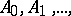whereis the initial matrix,, and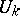is the matrix of the planar rotation which annihilates the off-diagonal entry of maximum modulus of the matrix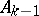. Here, if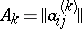,, the matrix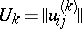differs from the identity matrix only by the entries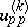,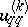,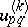,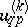. In the real case, whenis a symmetric matrix,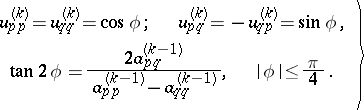(*)

In the complex case the relations (*) become insignificantly more complicated.

The sequence of matrices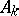converges to a diagonal matrix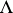, the rate of the convergence being asymptotically quadratic. The diagonal entries ofare approximate eigenvalues of, while the columns of the matrix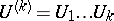are approximate eigenvectors.

The realization of this variant of the rotation method involves the choice of the off-diagonal matrix entry of maximum modulus at each step. The realization of this operation on a computer requires considerable computational labour.

There exist other variants of the rotation method, which are more effective in this respect: the cyclic rotation method, the rotation method with a barrier, and the rotation method with selection of an optimal element.

In the cyclic rotation method the pairs of indices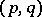of the entry that has to be annihilated cyclically run through all the upper-diagonal locations. The drawback of this method is that it may be necessary to carry out a large number of ineffective rotations that annihilate small off-diagonal entries.

This disadvantage is partly eliminated in the rotation method with a barrier, which involves the introduction of a sequence of numberscalled barriers, which monotonically decreases towards zero. During the cyclic review of indicesonly those off-diagonal entries with modulus larger thanare annihilated. In the following stage, after all the off-diagonal entries have become smaller than, the barrier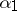is replaced by the barrier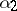and the process is continued. Application of this variant of the rotation method in practical work involves several difficulties, concerning the choice of an optimal barrier.

The method which is best suited for use in computational practice is the rotation method with selection of an optimal element . In this method the pairs of indicescorrespond to the almost-maximal entry and are so chosen thatis the number of a row with maximal Euclidean length, andis the number of a column of an off-diagonal entry of maximum modulus in the-th row. Since the rows of the matrix, except for the-th and-th row, do not vary in length at any stage of the process, the choice of the indicesdoes not significantly increase the computational labour involved. The entire theory of the classical rotation method is fully applicable to this modification .

The difference formulas of the rotation method which are used to calculate (*) ensure the convergence of the process of the rotation method under practical conditions of computer arithmetic, and also ensure highly accurate values of both eigenvalues and eigenvectors .

How to Cite This Entry:
Rotation method. Encyclopedia of Mathematics. URL: http://encyclopediaofmath.org/index.php?title=Rotation_method&oldid=15073
This article was adapted from an original article by G.D. Kim (originator), which appeared in Encyclopedia of Mathematics - ISBN 1402006098. See original article